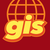# How to use geometry.distance in Python API

2128
3
04-09-2019 10:21 AMOccasional Contributor

I am trying to measure the distance between two points using the arcgis.geometry.distance function.

However, I get this error:

`RuntimeError: Unable to complete operation. The operation cannot be performed on a non-simple geometry. (Error Code: 400)`

My code is:

``````# Calculate distance
for index, values in cc_df.iterrows():
cap_point = values['SHAPE']  # A point object
print(type(cap_point), " geometry 1 type")
cap_stid = values['Study_ID']  # (string)

for c in dist_clm:  # A list of columns
moi = c[5:]  # The specific column
moi = moi[5:]  # A part of the column name used to acess the 'SHAPE' value in a dictionary
r_shp = rel_dict[cap_stid][moi]['shp']  # the 'SHAPE' column of a data frame
print(type(r_shp), " geometry 2 type")  # Printing the data type as part of testing
r_wkid = rel_dict[cap_stid][moi]['shp']['spatialReference']['wkid']  # The WKID (spatial refrence)

d = arcgis.geometry.distance(r_wkid, cap_point, r_shp, "METERS",
False))‍‍‍‍‍‍‍‍‍‍‍‍‍‍‍‍‍‍‍‍‍‍‍‍‍‍‍‍‍‍``````

It is basically extracting a point geometry from a dictionary and measuring the distance to another point geometry in a SPED. (Or that is what it is supposed to do)

The output is:

`<class 'arcgis.geometry._types.Point'>  geometry 1 type <class 'arcgis.geometry._types.Point'>  geometry 2 type`
`Unable to complete operation. The operation cannot be performed on a non-simple geometry.`

Are point objects not simple geometry?

The documentation on this is very sparse and I can't find any examples.

Here is a link to the documentation: arcgis.geometry module — arcgis 1.6.0 documentation

Any insight would be helpful. Thanks

arcgis 1.5.3

pandas 0.24.2

1 Solution

Accepted SolutionsOccasional Contributor III

Hi Ben,

For info on what Distance Units should be refer to this page: Distance—ArcGIS REST API: Services Directory | ArcGIS for Developers which branches off into:

It seems the function fails when you supply the distance unit directly (this might constitute a defect). However, it works when you store it in a variable like so:

``````from arcgis import geometry

sr = 102100
distance_unit = 9001

geom1 = {'x': -7703308.762905646, 'y': -2937284.4374484867,
'spatialReference': {'wkid': 102100}}
geom2 = {'x': 13614373.72405075, 'y': 2571663.047185693,
'spatialReference': {'wkid': 102100}}

pt1 = geometry.Point(geom1)
pt2 = geometry.Point(geom2)

d = arcgis.geometry.distance(sr, pt1, pt2, distance_unit, False)
print(d)‍‍‍‍‍‍‍‍‍‍‍‍‍‍‍``````

The result is:

{'distance': 22017994.663527936}

Kind regards,

Earl

3 RepliesOccasional Contributor

Not sure if this is helpful, but would your data fall into any of these categories?

https://support.esri.com/en/technical-article/000007177Occasional Contributor

Thanks for the speedy response Dylan.

It is a point feature so only the "Null Geometry" would apply. However, in exploring that possibility I found that deleting the distance units got rid of the error.  I set geodesic to True to get meters.

``arcgis.geometry.distance(r_wkid, cap_point, r_shp, geodesic=True)‍``

All the units I tried caused the error so it  must be something to do with that element.

geodesic will work find for my current application but it would be nice to know why planar with units defined didn't work.

Thanks,

Ben

```

```Occasional Contributor III

Hi Ben,

For info on what Distance Units should be refer to this page: Distance—ArcGIS REST API: Services Directory | ArcGIS for Developers which branches off into:

It seems the function fails when you supply the distance unit directly (this might constitute a defect). However, it works when you store it in a variable like so:

``````from arcgis import geometry

sr = 102100
distance_unit = 9001

geom1 = {'x': -7703308.762905646, 'y': -2937284.4374484867,
'spatialReference': {'wkid': 102100}}
geom2 = {'x': 13614373.72405075, 'y': 2571663.047185693,
'spatialReference': {'wkid': 102100}}

pt1 = geometry.Point(geom1)
pt2 = geometry.Point(geom2)

d = arcgis.geometry.distance(sr, pt1, pt2, distance_unit, False)
print(d)‍‍‍‍‍‍‍‍‍‍‍‍‍‍‍``````

The result is:

{'distance': 22017994.663527936}

Kind regards,

Earl